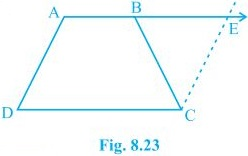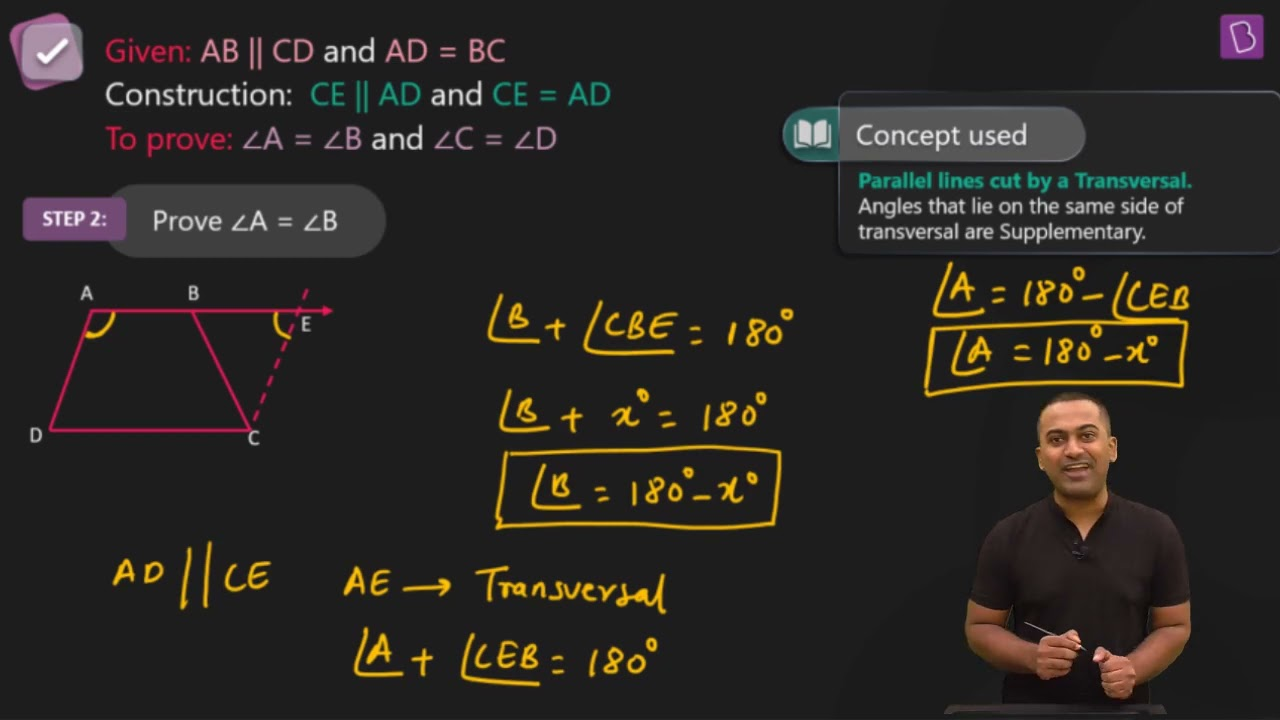# ABCD is a trapezium in which AB || CD and AD = BC (see Fig.) . Show that (i) ∠A = ∠B (ii) ∠C = ∠D (iii) ΔABC ≅ ΔBAD (iv) diagonal AC = diagonal BD [Hint : Extend AB and draw a line through C parallel to DA intersecting AB produced at E.]The trapezium ABCD in which AB || CD and AD = BC.

Extended AB and draw a line through C parallel to DA intersecting AB produced at E

(i) ∠A = ∠B

CE = AD (Opposite sides of a parallelogram)

, BC = CE

⇒∠CBE = ∠CEB

also,

∠A+∠CBE = 180° (Angles on the same side of transversal and ∠CBE = ∠CEB)

∠B +∠CBE = 180° ( As Linear pair)

⇒∠A = ∠B

(ii) ∠C = ∠D

∠A+∠D = ∠B+∠C = 180° (Angles on the same side of transversal)

⇒∠A+∠D = ∠A+∠C (∠A = ∠B)

⇒∠D = ∠C

AB = AB (Common)

∠DBA = ∠CBA

(iv) diagonal AC = diagonal BD

Diagonal AC = diagonal BD by CPCT as ΔABC ≅ ΔBA.(30)(11)